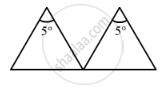Advertisement Remove all ads

# A Thin Prism of Crown Glass (μR = 1.515, μV = 1.525) and a Thin Prism of Flint Glass (μR = 1.612, μV = 1.632) Are Placed in Contact with Each Other. - Physics

Sum

A thin prism of crown glass (μr = 1.515, μv = 1.525) and a thin prism of flint glass (μr = 1.612, μv = 1.632) are placed in contact with each other. Their refracting angles are 5.0° each and are similarly directed. Calculate the angular dispersion produced by the combination.

Advertisement Remove all ads

#### SolutionFor crown glass, we have:-

Refractive index for red colour, μcr = 1.515

Refractive index for violet colour, μcv = 1.525

For flint glass, we have:-

Refractive index for red colour, μfr = 1.612

Refractive index for violet colour,μfv = 1.632

Refracting angle, A = 5°

Let:-

δc = Angle of deviation for crown glass

δf  = Angle of deviation for flint glass

As prisms are similarly directed and placed in contact with each other, the total deviation produced (δ) is given by

δ = δc + δf

= (μc - 1)A + (μf – 1)A

= (μc + μf – 2)A

For violet light, δv = (μcv + μfv – 2)A

For red light, δr = (μcr + μfr – 2)A

Now, we have:-

Angular dispersion of the combination:-

δv – δr = (μcv + μfv – 2)A – (μcr + μfr – 2)A

= (μcv + μfv – μcr – μfr) A

= (1.525 + 1.632 – 1.515 – 1.612)5

= 0.15°

So, the angular dispersion produced by the combination is 0.15°.

Is there an error in this question or solution?
Advertisement Remove all ads

#### APPEARS IN

HC Verma Class 11, 12 Concepts of Physics 1
Chapter 20 Dispersion and Spectra
Q 9 | Page 443
Advertisement Remove all ads

#### Video TutorialsVIEW ALL 

Advertisement Remove all ads
Share
Notifications

View all notifications

Forgot password?